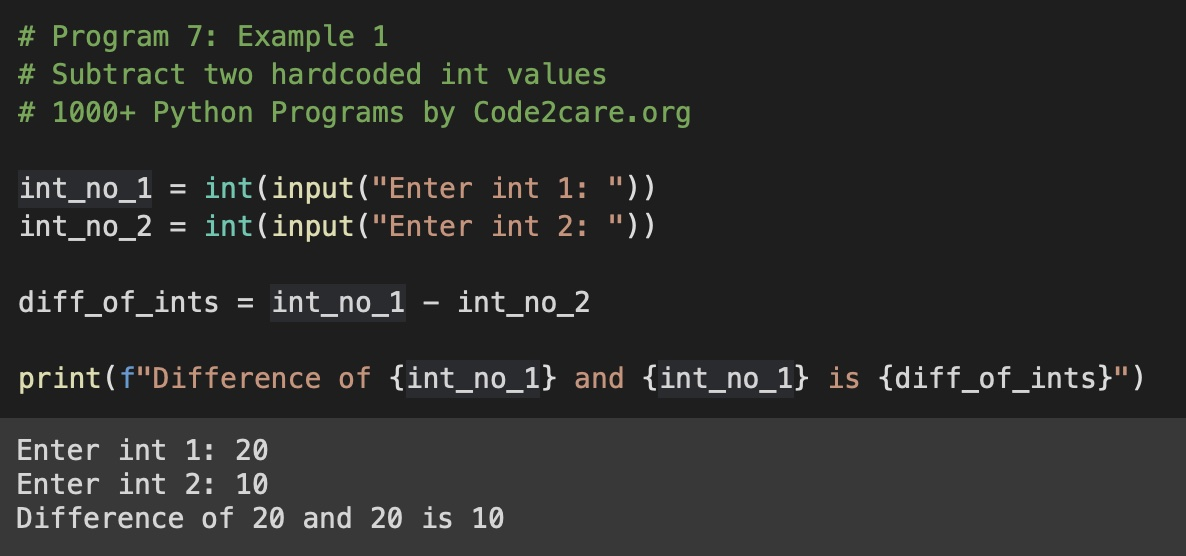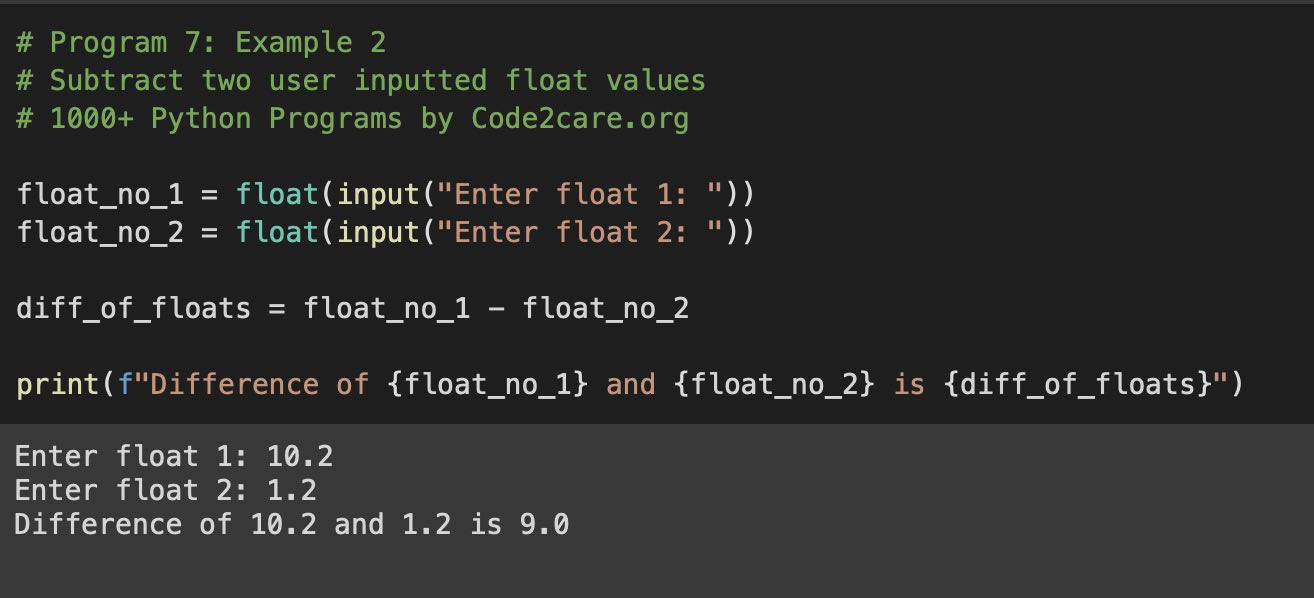# Program 7: Find Difference of Two Numbers - 1000+ Python Programs

Question 7)

Write a program in Python to find the difference between two numbers.
Write a program in Python to subtract two numbers.
Write a program to subtract two numbers by taking user input using Python Programming.
Program to find the difference between two integer values in Python.

## Code Example 1: Subtract two Integers

``````# Program 7: Example 1
# Subtract two hardcoded int values
# 1000+ Python Programs by Code2care.org

int_no_1 = int(input("Enter int 1: "))
int_no_2 = int(input("Enter int 2: "))

diff_of_ints = int_no_1 - int_no_2

print(f"Difference of {int_no_1} and {int_no_1} is {diff_of_ints}")``````
Output:

Enter int 1: 20
Enter int 2: 10
Difference of 20 and 20 is 10## Code Example 1: Difference between two user inputted floats

``````# Program 7: Example 2
# Subtract two user-inputted float values
# 1000+ Python Programs by Code2care.org

float_no_1 = float(input("Enter float 1: "))
float_no_2 = float(input("Enter float 2: "))

diff_of_floats = float_no_1 - float_no_2

print(f"The difference between  {float_no_1} and {float_no_2} is {diff_of_floats}")``````
Output:

Enter int 1: 10.2
Enter int 2: 1.2
The difference between 10.2 and 1.2 is 9.0## Explaination:

List of functions used,

1. print(): Built-in function to print a string on the console with f-strings formatting.
2. float(): Built-in function to convert String argument to float value.
3. input(): Built-in function to accept user input

### Steps:

1. Create a variable to hold first integer/float
2. Create a variable to hold a second integer/float
3. Create a third variable to hold the subtraction of two variables using the - (minus) operator.
4. Print the result using print() function.